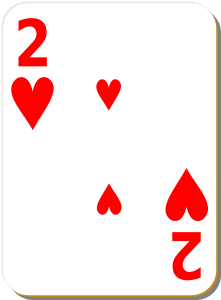# Definition of OddsOdds tell us how likely something is to happen.

To calculate the odds of something happening, you find the ratio of the number of ways that thing can occur to the number of ways it doesn't occur.

For example,

• The odds of drawing the 2 of hearts from a deck of $52$ playing cares are $1:51$.
• The odds of drawing a blue ball from a bag containing 3 blue balls and 4 red balls are $3:4$
• The odds of a coin landing tails up are $1:2$.

### Description

The aim of this dictionary is to provide definitions to common mathematical terms. Students learn a new math skill every week at school, sometimes just before they start a new skill, if they want to look at what a specific term means, this is where this dictionary will become handy and a go-to guide for a student.

### Audience

Year 1 to Year 12 students

### Learning Objectives

Learn common math terms starting with letter O

Author: Subject Coach
You must be logged in as Student to ask a Question.